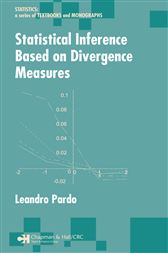# Statistical Inference Based on Divergence Measures

## Series: Statistics: A Series of Textbooks and MonographsCA\$67.77
###### ISBNs
• 9781420034813
The idea of using functionals of Information Theory, such as entropies or divergences, in statistical inference is not new. However, in spite of the fact that divergence statistics have become a very good alternative to the classical likelihood ratio test and the Pearson-type statistic in discrete models, many statisticians remain unaware of this powerful approach.

Statistical Inference Based on Divergence Measures explores classical problems of statistical inference, such as estimation and hypothesis testing, on the basis of measures of entropy and divergence. The first two chapters form an overview, from a statistical perspective, of the most important measures of entropy and divergence and study their properties. The author then examines the statistical analysis of discrete multivariate data with emphasis is on problems in contingency tables and loglinear models using phi-divergence test statistics as well as minimum phi-divergence estimators. The final chapter looks at testing in general populations, presenting the interesting possibility of introducing alternative test statistics to classical ones like Wald, Rao, and likelihood ratio. Each chapter concludes with exercises that clarify the theoretical results and present additional results that complement the main discussions.

Clear, comprehensive, and logically developed, this book offers a unique opportunity to gain not only a new perspective on some standard statistics problems, but the tools to put it into practice.
###### ISBNs
• 9781420034813